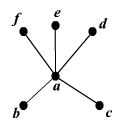# Degree of a Vertex

In graph theory , the degree of a vertex is the number of edges connecting it. In the example below, vertex $a$ has degree $5$ , and the rest have degree $1$ . A vertex with degree $1$ is called an "end vertex" (you can see why).